makeweight

Weighting function with monotonic gain profile

Description

makeweight is a convenient way to specify loop shapes, target gain profiles, or weighting functions for applications such as controller synthesis and control system tuning.

example

W = makeweight(dcgain,[freq,mag],hfgain) creates a first-order, continuous-time weight W(s) satisfying these constraints:

$\begin{array}{c}W\left(0\right)=\text{dcgain}\\ W\left(\text{Inf}\right)=\text{hfgain}\\ |W\left(j\cdot \text{freq}\right)|=\text{mag}\text{.}\end{array}$

In other words, the gain of W passes through mag at the finite frequency freq.

example

W = makeweight(dcgain,[freq,mag],hfgain,Ts) creates a first-order, discrete-time weight W(z) satisfying these constraints:

$\begin{array}{c}W\left(1\right)=\text{dcgain}\\ W\left(-1\right)=\text{hfgain}\\ |W\left({e}^{j\cdot \text{freq}\cdot \text{Ts}}\right)|=\text{mag}\text{.}\end{array}$

In other words, the gain of W passes through mag at the frequency freq. The frequency freq must satisfy 0 < freq < π/Ts.

example

W = makeweight(dcgain,[freq,mag],hfgain,Ts,N) uses an Nth-order transfer function with poles and zeros in a Butterworth pattern to meet the constraints. The higher the order N, the steeper the transition from low to high gain. To create a continuous-time higher order weighting function, use Ts = 0.

example

W = makeweight(dcgain,wc,hfgain,___) specifies the gain crossover frequency wc. This syntax is equivalent to setting [freq,mag] to [wc,1]. You can use this syntax with any of the previous input-argument combinations to create a continuous-time, discrete-time, or Butterworth weighting function.

Examples

collapse all

Create continuous-time weighting functions by specifying the low-frequency gain, high-frequency gain, and magnitude of the gain at some intermediate frequency.

For instance, create a weighting function with a gain of 40 dB at low frequency, rolling off to –20 dB at high frequency. Specify further that the gain is about 10 dB at 1 rad/s by putting these values in a vector [freq,mag]. Specify all the gains in absolute units.

Wl = makeweight(100,[1,3.16],0.1);

Create a weighting function with a gain of –10 dB at low frequency, rising to 40 dB at high frequency. Specify a 0 dB crossover frequency of 10 rad/s. To specify a 0 dB crossover frequency, you can use the crossover frequency as the second input argument instead of the vector [freq,mag].

Wh = makeweight(0.316,10,100);

Plot the magnitudes of the weighting functions to confirm that they meet the response specifications.

bodemag(Wl,Wh)
legend
grid on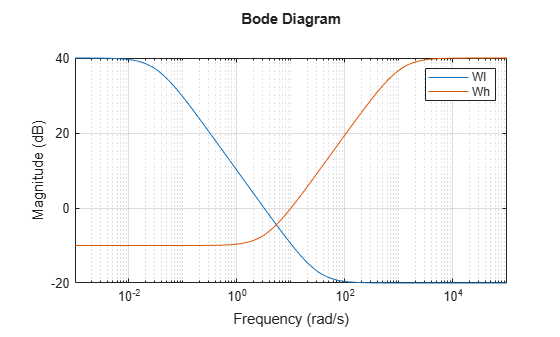Create a gain profile that rolls off at high frequency without flattening. Specify a gain of 40 dB at low frequency and a crossover frequency of 10 rad/s.

W = makeweight(100,[10 1],0);

Specifying a high-frequency gain of 0 ensures that the frequency response rolls off at high frequencies without leveling off. Plot the gain profile to confirm this shape.

bodemag(W)
grid on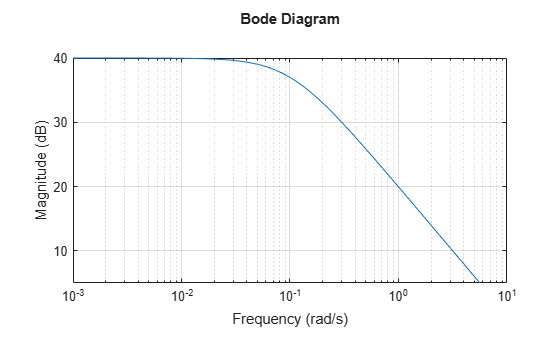Create discrete-time weighting functions by specifying the low-frequency gain, high-frequency gain, magnitude of the gain at some intermediate frequency, and sample time.

Create a weighting function with a sample time of 0.1 s. Specify a gain of 40 dB at low frequency, rolling off to –20 dB at high frequency. Specify further that the gain is about 10 dB at 0.01 rad/s. Provide all gains in absolute units.

Wl = makeweight(100,[0.01,3.16],0.1,0.1);

Create a weighting function with a gain of –10 dB at low frequency, rising to 40 dB at high frequency. Specify a 0 dB crossover frequency of 2 rad/s and a sample time of 0.1 s. To specify a 0 dB crossover frequency, you can use the crossover frequency as the second input argument instead of the vector [freq,mag].

Wh = makeweight(0.316,2,100,0.1);

Plot the magnitudes of the weighting functions to confirm that they meet the response specifications.

bodemag(Wl,Wh)
grid on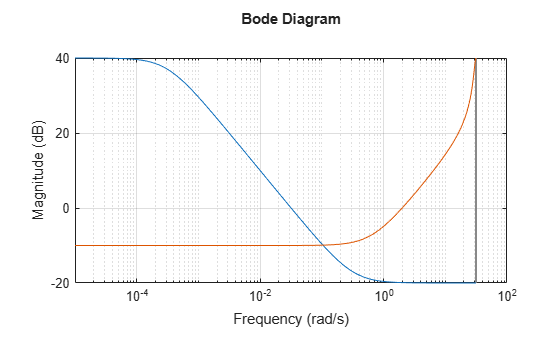The high-frequency leveling of Wh is distorted due to the proximity of its crossover frequency to the Nyquist frequency.

By default, makeweight creates first-order weighting functions. If you want a sharper transition between the low-frequency and high-frequency gains, you can specify the order with the last input argument. For instance, suppose you want to create a weighting function with a sample time of 0.1 s. The function has a gain of –10 dB at low frequency, rising to 40 dB at high frequency. Additionally, the gain passes through 6 dB at 1 rad/s. For comparison, create both a third-order and a first-order function with these specifications.

W3 = makeweight(0.316,[1 2],100,0.1,3);
W1 = makeweight(0.316,[1 2],100,0.1);
bodemag(W3,W1)
legend('location','northwest')
grid on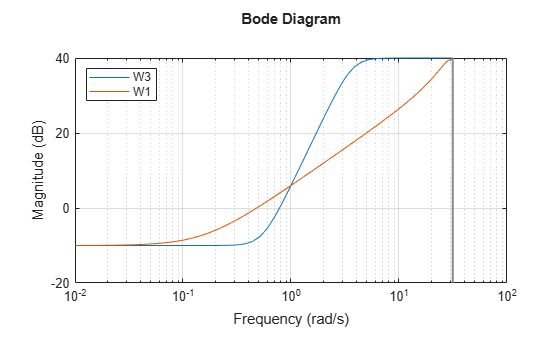For the first-order function, the high-frequency leveling is distorted due to the proximity of its crossover frequency to the Nyquist frequency. Using a sharper, higher-order transition ensures that the function has leveled out before reaching the Nyquist frequency.

To create continuous-time weighting functions of higher order, set Ts = 0. For instance, create continuous-time weighting functions with the same gain specifications as W1 and W3.

W3c = makeweight(0.316,[1 2],100,0,3);
W1c = makeweight(0.316,[1 2],100);
bodemag(W3c,W1c)
legend('location','northwest')
grid on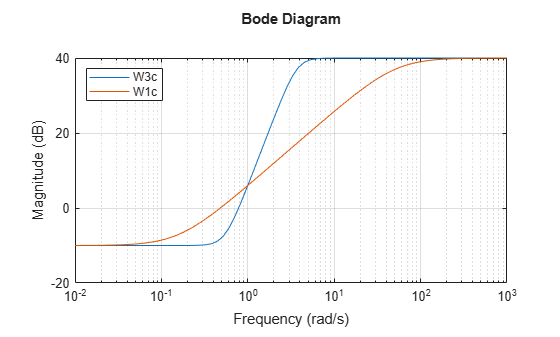Input Arguments

collapse all

Low-frequency gain of the weighting function, specified as a real scalar value. Express the gain in absolute units. For example, to specify a low-frequency gain of 20 dB, set dcgain = 10.

The low-frequency gain, high-frequency gain, and magnitude must satisfy:

• |dcgain| > mag > |hfgain| for a low-pass weight

• |dcgain| < mag < |hfgain| for a high-pass weight

Target magnitude and corresponding frequency, specified as a two-element vector. You specify where the gain of W transitions between the low-frequency and high-frequency values by specifying a target magnitude at a particular frequency. For instance, if you set [freq,mag] = [10,0.1], then the magnitude of W passes through 0.1 (–10 dB) at a frequency of 10 rad/s. Similarly, setting [freq,mag] = [5,1] specifies a 0 dB (unit gain) crossover frequency of 5 rad/s.

The low-frequency gain, high-frequency gain, and magnitude must satisfy:

• |dcgain| > mag > |hfgain| for a low-pass weight

• |dcgain| < mag < |hfgain| for a high-pass weight

High-frequency gain of the weighting function, specified as a real scalar value. Express the gain in absolute units. For example, to specify a high-frequency gain of –20 dB, set dcgain = 0.1.

The low-frequency gain, high-frequency gain, and magnitude must satisfy:

• |dcgain| > mag > |hfgain| for a low-pass weight

• |dcgain| < mag < |hfgain| for a high-pass weight

Sample time of discrete-time weighting function, specified as a nonnegative scalar value or –1. A positive value sets the sample time in seconds. The special value –1 creates a discrete-time state-space model with an unspecified sample time.

Setting Ts = 0 creates a continuous-time weighting function. This value is useful when you want to create higher order continuous-time transfer functions using the N input argument. For an example, see Higher Order Weighting Functions.

Order of weighting function, specified as a positive integer. makeweight uses an Nth-order transfer function with poles and zeros in a Butterworth pattern to meet the specified gain constraints. The higher the order N, the steeper the transition from low to high gain.

Crossover frequency of the weighting function in radians/second, specified as a positive scalar value. Using the input argument wc is equivalent to using [freq,mag] = [wc,1].

For discrete-time weighting functions, the crossover frequency must satisfy wc*Ts < π.

Output Arguments

collapse all

Weighting function, returned as a state-space (ss) model. For continuous-time weighting functions, the response of W satisfies the following:

$\begin{array}{c}W\left(0\right)=\text{dcgain}\\ W\left(\text{Inf}\right)=\text{hfgain}\\ |W\left(j\cdot \text{freq}\right)|=\text{mag}\text{.}\end{array}$

For discrete-time weighting functions, the response of W satisfies the following:

$\begin{array}{c}W\left(1\right)=\text{dcgain}\\ W\left(-1\right)=\text{hfgain}\\ |W\left({e}^{j\cdot \text{freq}\cdot \text{Ts}}\right)|=\text{mag}\text{.}\end{array}$